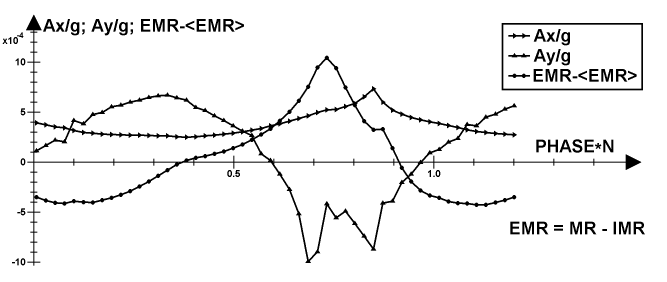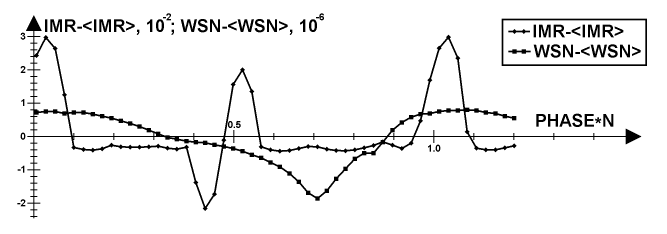# Vibrations reduction system (VRS)

A comfortable flight requires a low level of vibrations of the rotor. Entire modeling of the aircraft was used to obtain appropriate data samples for analyzing such vibrations. Five components can be selected for the analysis of these vibrations. The first two are the same as I presented for the analysis of the "wired wings" simulations. These are the horizontal and vertical components of the acceleration of the entire aircraft, normalized by gravitational acceleration at the ground level. The third component is the deviation of the external moment ratio EMR or shaft moment ratio. This deviation is calculated as the difference between the instantaneous value and the value averaged over all analyzed samples. The fourth component is the deviation of IMR. And the fifth component is the deviation of WSN. Also, it can be understood that for this analysis only samples are enough that lay in the time range that corresponds to the range of positions between any two neighbor wings or a bit more. For N wings, this periodic time range is the N-th fraction of the entire rotor phase, and so I refer to the time inside it as a minor phase. Also, I consider that it varies globally from zero to N, instead of from zero to one periodically, as this is better for analysis. And so, I extracted such data from the result of the flight simulation for the cruise flight and prepared plots for this. A plot for the first three mentioned components is represented below.This plot exposes quite low deviations for the vertical acceleration and EMR. In the case of referencing the EMR at the radius of the rotor, both their impacts will be below 0.001g on the amplitude basis. And also, they are in counter phase, so some compensation will exist, since the center of gravity of the aircraft is located some under the forward side of the rotor. And for the horizontal acceleration, these variations are much lower.The plot above represents the two remained components, which are exactly synchronized by their common horizontal axes of the minor phase with the previous plot. This plot exposes significantly high deviations for IMR, which have an amplitude of about 0.03g at the radius of the rotor and a spectrum of high harmonics. Also, deviations of WSN, presented here, are low, with an amplitude below 0.000002, or 0.2mm/s if a normalizing speed of 100 m/s is used. Also, they are synchronized with EMR. The vibrations from IMR can be reduced simply by using a symmetrical airfoil. But there is another way. This can be performed by an active vibration reduction system VRS, which poses special patterns of additional current in the coils of the electric engine of the rotor to compensate for the vibrations propagating through the stationary elements of the rotor. These patterns should be synchronized with the minor phase of the rotor. In addition, the low level of these WSN variations provides sufficient margin for this. Also, this VRS will be more simplified if the number of the wings is a common divider of the number of poles of the engine. Also, such a pattern shouldn't follow on straightforward manner from the presented plots, since it depends on the actual mechanics properties of all the tiers of the aircraft near the rotor's vicinity and on particular flight operations. And so, it can only be obtained experimentally or through detailed modeling.

The active VRS can be implemented as an extension of the central computer mediated in-flight management. The VRS applies compensating patterns of currents to the coils of both engines by a pattern generator connected to both power circuits. The pattern generator plays a particular active pattern, which is scaled in time using a minor phase signal. Particularly, the minor phase signal can be synchronized with the phase powering of these engines for a particular number of poles in the rotors of these engines. In another case a separated minor phase sensor extracts this minor phase signal from both rotors. The central computer can manage this pattern generator through loading this active pattern from a pattern store, dependently on a particular flight operation or a load state. Also, this pattern generator can be implemented as a part of the engine controller, especially in the case of synchronizing the minor phase signal with the phase powering of the engines.